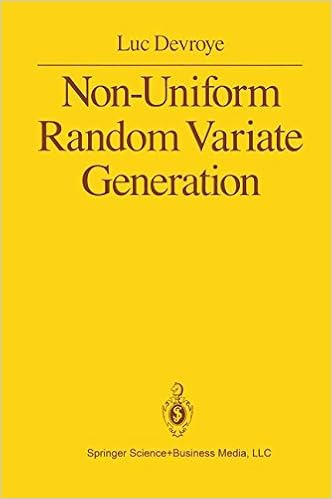## ratio-of-uniforms [#3]Being still puzzled (!) by the ratio-of-uniform approach, mostly failing to catch its relevance for either standard distributions in a era when computing a cosine or an exponential is negligible, or non-standard distributions for which computing bounds and boundaries is out-of-reach, I kept searching for solutions that would include unbounded densities and still produce compact boxes, as this seems essential for accept-reject simulation if not for slice sampling. And after exploring some dead-ends (in tune with running in Venezia!), I came upon the case of the generalised logistic transform$h(\omega)=\omega^a/(1+\omega^a)$

which ensures that the [ratio-of-almost-uniform] set I defined in my slides last week$\mathfrak{H}=\left\{(u,v);\ 0\le u\le h(f(v/g(u))\right\}$

is bounded in u. Since the transform g is the derivative of the inverse of h (!),$g(y)=a^{-1}y^{(1-a)/a}/(1-y)^{(1-3a)/a}$

the parametrisation of the boundary of H is$u(x)=f(x)^a/(1+f(x)^a)\ v(x)=a^{-1}xf(x)^{(a-1)/a}(1+f(x)^a)^2$

which means it remains bounded if (a) a≤1 [to ensure boundedness at infinity] and (b) the limit of v(x) at zero [where I assume the asymptote stands] is bounded. Meaning$\lim_{x\to 0} xf(x)^{2a+1/a-1}<\infty$

Working a wee bit more on the problem led me to realise that resorting to an arbitrary cdf Φ instead of the logistic cdf could solve the problem for most distributions, including all Gammas! Indeed, the boundary of H is now$u(x)=\Phi(f(x))^a\ v(x)=a^{-1}xf(x)^{(a-1)/a}/\varphi(f(x))$

which means it remains bounded if φ has very heavy tails, like 1/x². To handle the explosion when x=0. And an asymptote itself at zero, to handle the limit at infinity when f(x) goes to zero.

### 2 Responses to “ratio-of-uniforms [#3]”

1.Han Oostdijk Says:

Unreadable formulas (maybe related to the age of my eyes)

•xi'an Says:

Sorry, they look fine on my computer, but I presume mileage may vary depending on the device!

This site uses Akismet to reduce spam. Learn how your comment data is processed.